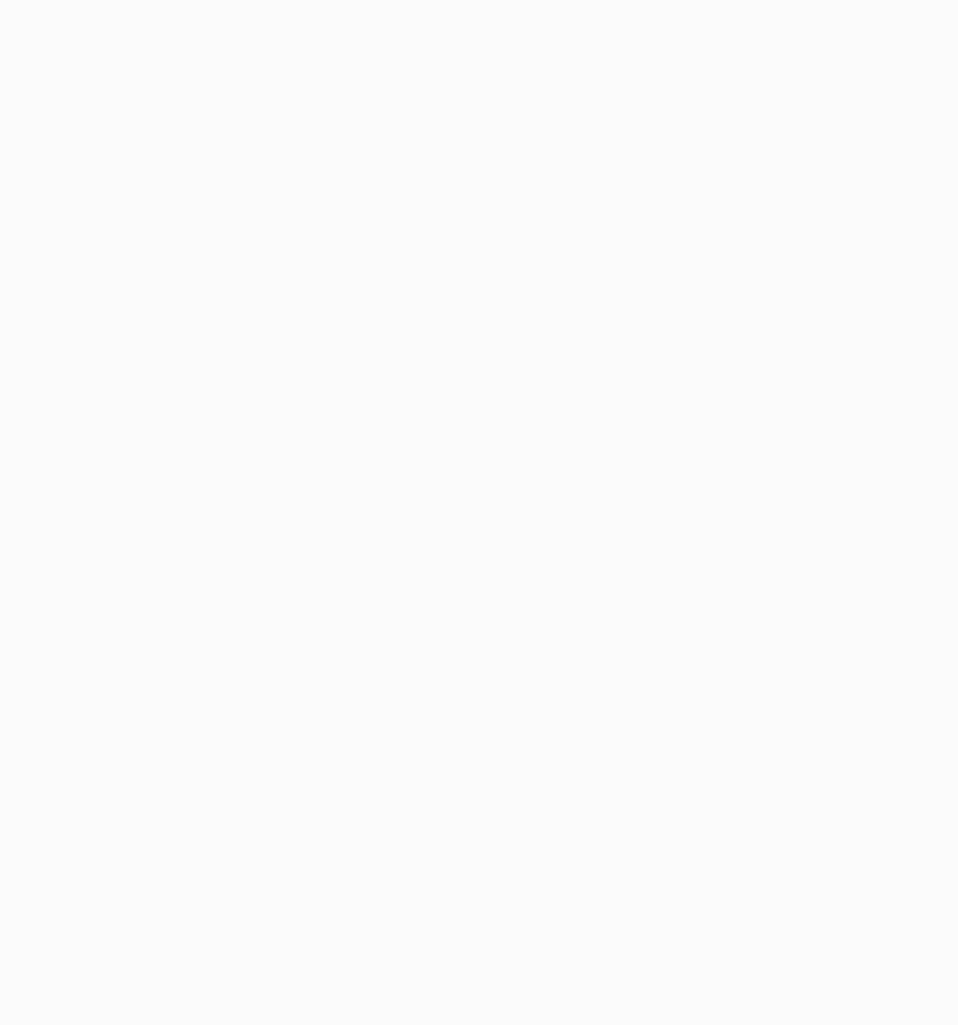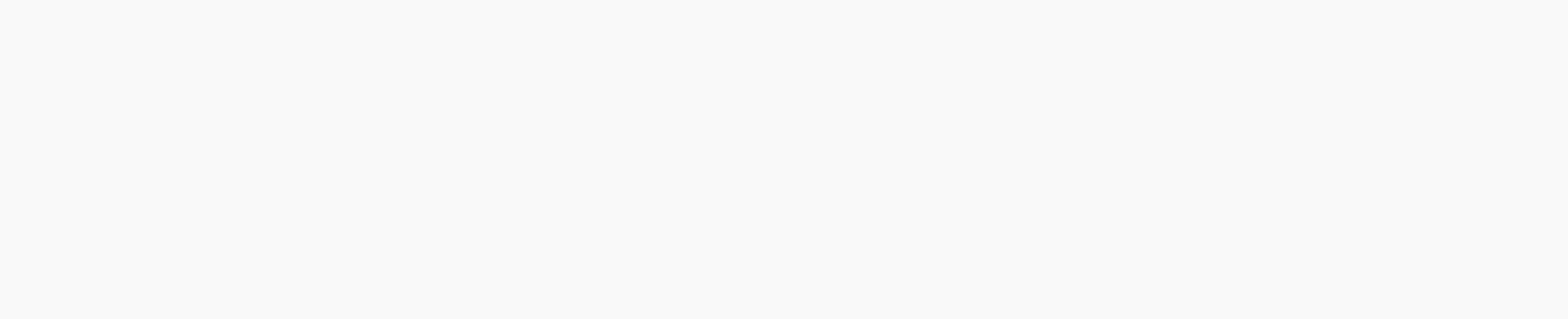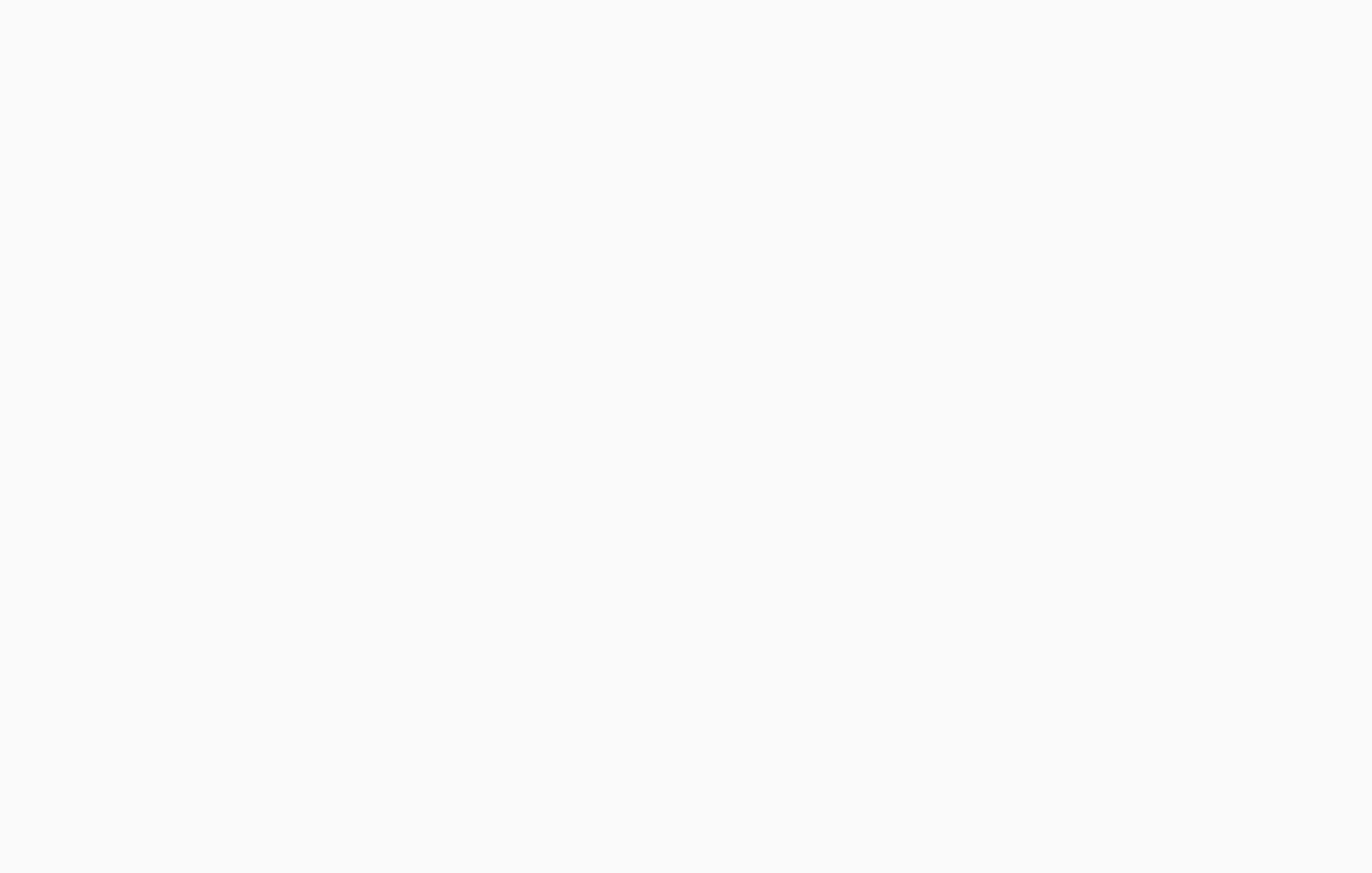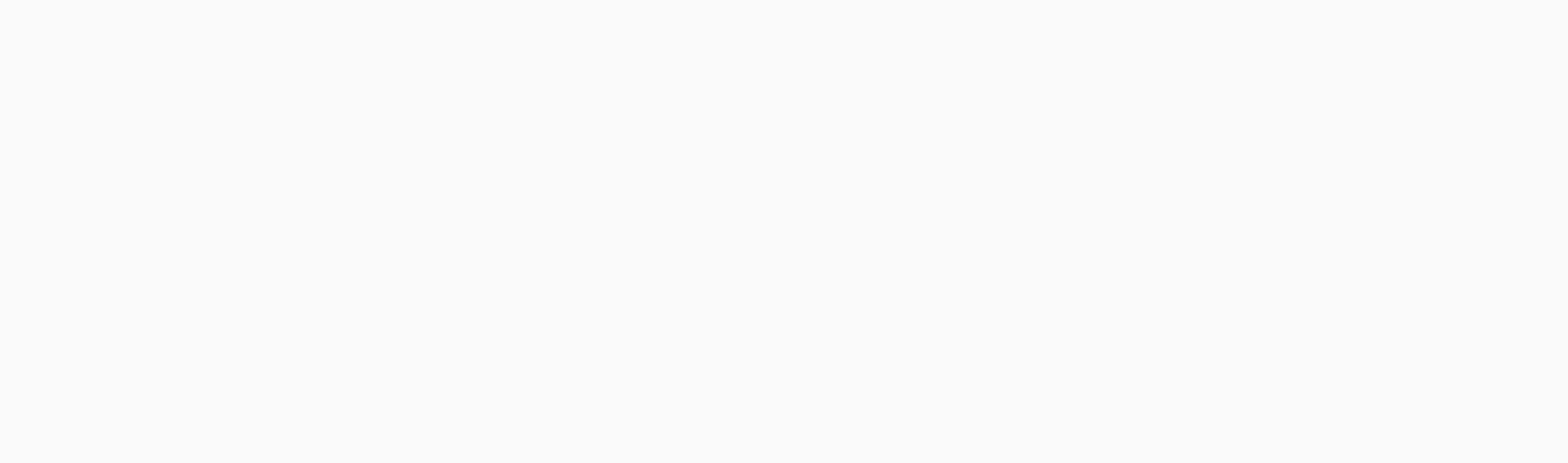# 6 Refraction of Light Exercise Solutions Science 1 – Maharashtra board

6 Refraction of Light Exercise, Maharashtra Board Class 10 Science Solutions Part 1 Chapter 6 Refraction of Light Exercise, 6 Refraction of Light Class 10 question and answers, 6 Refraction of Light class 10 important questions and answers PDF

# 6 Refraction of Light Exercise Solutions Science 1 – Maharashtra board

## Question No. 1]

### Fill in the blanks and explain the completed statements:

a. Refractive index depends on the………….of light.

Refractive index depends on the velocity of light.

It is an experimental fact. (There is no question of explanation.)

b. The change in…………of light rays while going from one medium to another is called refraction.

The change in the direction of propagation of light rays while going from one medium to another is called refraction.

This is definition of refraction. It is assumed that the ray of light passes obliquely from one medium to another. (There is no question of explanation.)

6.Refraction of Light

6 Refraction of Light Exercise

## Question No.2]

### Prove the following statements:

a. If the angle of incidence and angle of emergence of a light ray falling on a glass slab are i and e respectively, prove that i = e.

In the following figure, SR || PQ and NM is the refracted ray. Hence, r = i1.

Now gna = sin i/sin r and ang = sin i1/ sin e.As r = i1, it follows that sin i = sin e
∴ i = e.

b. A rainbow is the combined effect (an exhibition) of the refraction, dispersion, and total internal reflection of light.

(OR)

With a neat labelled diagram, explain how the formation of rainbow occurs.

(1) The formation of a rainbow in the sky is a combined result of refraction, dispersion, internal reflection and again refraction of sunlight by water droplets present in the atmosphere after it has rained.Formation of a rainbow

Here, for simplicity only violet and red colours are shown. The remaining five colours lie between these two.

(2) The sunlight is a mixture of seven colours: violet, indigo, blue, green, yellow, orange and red. After it has stopped raining, the atmosphere contains a large number of water droplets. When sunlight is incident on a water droplet, there is (i) refraction and dispersion of light as it passes from air to water (ii) internal reflection of light inside the droplet and (iii) refraction of light as it passes from water to air.

(3) The refractive index of water is different for different colours, being maximum for violet and minimum for red. Hence, there is dispersion of light (separation into different colours) as it passes from air to water. [ See above Figure for reference.]

(4) The combined action of different water droplets, acting like tiny prisms, is to produce a rainbow with red colour at the outer side and violet colour at the inner side. The remaining five colours lie between these two.

The rainbow is seen when the sun is behind the observer and water droplets in the front.

Refraction of Light Class 10 question and answers

## Question No.3]

### Mark the correct answer in the following questions :

A. What is the reason for the twinkling of stars?

(i) Explosions occurring in stars from time to time

(ii) Absorption of light in the earth’s atmosphere

(iii) Motion of stars

(iv) Changing refractive index of the atmospheric gases

(iv) Changing refractive index of the atmospheric gases.

B. We can see the Sun even when it is little below the horizon because of

(i) reflection of light

(ii) refraction of light

(iii) dispersion of light

(iv) absorption of light

(ii) refraction of light

C. If the refractive index of glass with respect to air is 3/2, what is the refractive index of air with respect to glass?

(i) 1/2

(ii) 3

(iii) 1/3

(iv) 2/3

(iv) 2/3

6 Refraction of Light Exercise

## Question No.4]

### Solve the following examples:

a. If the speed of light in a medium is 1.5 × 108 m/s, what is the absolute refractive index of the medium?

solution: Data: v = 1.5 × 108 m/s,
c = 3 × 108 m/s, n = ?This is the absolute refractive index of the medium.

b. If the absolute refractive indices of glass and water are 3/2 and 4/3 respectively, what is the refractive index of glass with respect to water?